ISSN: 0970-938X (Print) | 0976-1683 (Electronic)

# Biomedical Research

An International Journal of Medical Sciences

Research Article - Biomedical Research (2017) Volume 28, Issue 11

## Designing wearable joystick and performance comparison of EMG classification methods for thumb finger gestures of joystick control

Cemil Altin* and Orhan ER

Department of Electrical and Electronics Engineering, Faculty of Engineering and Architecture, Bozok University, Yozgat, Turkey

*Corresponding Author:
Cemil Altin
Department of Electrical and Electronics Engineering
Faculty of Engineering and Architecture, Bozok University, Turkey

Accepted date: March 09, 2017

Visit for more related articles at Biomedical Research

### Abstract

Surface electromyography is often used in the control of some devices, especially in the control of the prostheses. In this work, surface electromyography (EMG) is used to perform the function of a drone’s remote control joystick. To design a wearable joystick and select the best classification algorithm, the system first learns thumb finger movements when moving a joystick forward, backward, right, left, and neutral, and then classifies new thumb movements as learned by different classifiers. The data set was obtained with our own EMG device. Autoregressive (AR) modelling, mean absolute deviation, waveform length, entropy, integrated absolute amplitude, mode, percentile and interquartile are used as the feature extraction. Various classification algorithms such as neural networks (NN), discriminant analysis (DA), k-nearest neighbour (KNN), support vector machine (SVM) and Naive Bayes have been used and compared. The performance of each classifier algorithm is defined as the ratio of correctly classified samples to the total number of samples. According to experimental results, LDA gives the highest correct classification ratio and KNN is the most robust classifier.

## Keywords

Biomedical measurement, Biomedical signal processing, Classification algorithms, Feature extraction, Gesture recognition.

## Introduction

The problem of drone control with a remote control handheld in a hazardous environment, and especially the special conditions in military operations, has led us to design a handsfree remote controller. In order for a drone to be controlled by a conventional remote control handheld, both left and right hands must be used for holding and control. In the proposed system, three disposable EMG electrodes are attached to the arm to capture the function of the joystick on the handheld, so that a handheld machine does not need to be held and the hands free. This will allow the drone user to use his/her hand to use extra objects such as weapons during drone control. In the literature wearable joystick is studied by different researchers by different techniques. Most of these studies concern gloves that recognize hand movements. These gloves use sensors such as electro-optic, piezo-resistive, Hall Effect, pressure, accelerometer, gyroscope to identify hand movements. Some of these gloves are Sayre Glove, MIT LED Glove, Data Glove, Power Glove, P5 Glove, Space Glove, Cyber Glove . However, these gloves use the user's wrist movements or hand movements. But our wearable control joystick uses only thumb motions to perform the function of the control joysticks and does not use any sensor technology except for simple EMG electrodes for getting EMG signals. The disadvantages of glove systems are fragility, complexity, having a large number of cables, and being costly etc., . In addition to the glove systems, there is another study about wearable joystick which is studied by Saha in the Virginia Polytechnic Institute and State University. The Saha’s wearable joystick uses mechanomyographic (MMG) signals to recognize the thumb movements . Mechanomyography is a low-frequency vibration produced by the muscles and Mechanomyography is the mechanical feature produced by the skeletal muscle . Our study is different from the work of Saha in the method of acquiring muscle activity. In this study, EMG is used as a method of acquiring muscle activity due to its unbreakable, simplicity, fewer cables, the lack of additional sensors and the advantages of being cheaper compared to glove systems. In addition, electromyography has the advantages of being a gold standard in acquiring muscle activity compared to MMG, and not being sensitive to noise. Due to the advantages mentioned, EMG was used in the wearable joystick design.

## Materials and Methods

Data acquisition and processing materials

In this work EMG signals are captured by OL?MEX shield- EKG-EMG platform seen in Figure 1. This is a single channel, low cost and open source device. The reason for choosing this device is that it is open source and low cost. Open source devices are more suitable for application based study. Because the user can easily process the data using only one computer and some traditional programs like MATLAB or C++. The electrodes are Ag/AgCl solid adhesive pre-gelled type.

Figure 1: MATLAB R2009b is used as signal processing tool on a 2.3 GHz Dual Core laptop.

EMG data acquisition

EMG signals are generated by ion exchange of muscle membranes and are detected with the aid of electrodes . It is important to place the electrodes on the proper muscle to get the proper signal for the movements. Major muscles related with the thumb gestures are Abductor Pollicis Longus, Extensor Pollicis Longus and Extensor Policis Brevis. Electrode placement must be made properly and the repeatability of the electrode placement in different sessions or on different users should be the same. Because both the electrode placement and the repeatability of thumb movements affect the accuracy of the system. Electrode placement and proximity of the test motion to the training motion affects the accuracy of the system.

EMG signal filtering

Amplitude of the EMG signals varies between 0 to 10 mV (peak to peak) and 0 to 1.5 mV (rms). Useful part of the signal is available between 0 to 500 Hz ranges . But in this range EMG signal has lots of different noises so filtering the EMG signal is essential. The most existing noises in the EMG are AC mains power line noise and movement artifacts. So, high pass filter is required in order to compensate low frequency movement artifact (typically<10 Hz) . AC mains power line noise is also compensated via Notch filter. Also if desired low pass filter can be used to eliminate high-frequency ingredients to avoid signal aliasing.

Feature extraction

Feature extraction is required to identify EMG data. In this work, five hand gestures will be identified. These are forcing the thumb backward for the back joystick command, forcing the thumb to the right side for the right joystick command, forcing the thumb to the left side for the left joystick command, forcing the finger forward for the forward joystick command, and holding the neutral for the no movement command. This is shown in Figure 2.

Figure 2: Thumb gestures both remote controller and EMG acquisition system. A) Right B) Left C) Forward D) Backward E) No movement.

Thumb muscles will produce different signals in 5 different positions, so we will get 5 different EMG data. These differences in 5 different positions should be determined statistically or mathematically. This is feature extraction.

Autoregressive coefficients

AR modeling is acquisition of an equation which well fits to the signal and represents the signal. AR modelling estimates the signal data points according to the previous data points.→(1)

Here, p is degree of the AR model, x[n] is data signal which composed of n data points, ak is real valued AR coefficients and e[n] is white noise term which is independent from previous samples .

Mean absolute deviation

Mean absolute deviation is mean of the absolute deviations of data subjects from their mean .→(2)

Here, x [n] is data signal which composed of n data points and μ is the mean of whole signal.

Waveform length

Waveform length is a computation of complexity of the signal. It is identified as cumulative length of the waveform over the time range.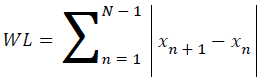→(3)

Integrated absolute amplitude

Integrated absolute amplitude is the sum of absolute of each data point. This feature is also parallel to mean function when the result is divided to data point number.→(4)

Wavelet entropy

Shannon entropy and log energy entropy types are used. The Shannon entropy is a functional of a probability distribution that is, it takes a probability distribution as an entry and converts to a number . Shannon entropy is also a standard of the randomness of a distribution, and plays a vital role in statistics, information theory and coding theory . The definition of Shannon entropy is as follows .→(5)

Ejn is the wavelet energy spectrum at scale j and instant n and its definition is like below.→(6)→(7)

Here Dj (n) is a wavelet coefficient. Log energy entropy is defined like below.→(8)

Mode

This feature helps us to find most frequent data in the EMG signal. Mode is dependent to the size of the data and interval of two points. So each data should be same length and interval.

Percentile

This feature is needed for acquiring useful summary of the EMG signal pattern. Here, the signal is divided into 100 points from the beginning of the signal to end of the signal. Then the successive data points are linked to each other using linear interpolation. At the end we can get whatever data points we want. For example 2.5, 25, 50, 75 and 97.5 combinations is one of the useful summaries of the data. Here 50 is the median value of our data.

Interquartile

Interquartile is like percentile, but it divides the data into not 100 equal parts it divides the data only 4 equal parts. Interquartile is needed to measuring the dispersion or spread of the data. Interquartile is difference between the first and third quartile or difference between the 75th and the 25th percentiles of the data.

Classification algorithms

After different 5 thumb EMG signals are identified by feature extraction methods, they are classified by classifier according to feature similarity. There are many classifiers in the literature, and choosing the appropriate classifier increases system performance by up to 20%. In this study Artificial Neural Networks, K-Nearest Neighbour, Support Vector Machines, Discriminant Analysis, and Naïve Bayes algorithms are used as classifier and compared. In the following subsections principles of these algorithms are explained.

K nearest neighbour

KNN classification algorithm is very usable for classification works. The algorithm is simple and efficient. In K nearest neighbour algorithm, the distance of the test sample to all the other samples of other groups is calculated. When the minimum distance is found the sample is belong to that group.

All of the distances are calculated by Euclidian theorem generally.→(9)

According to Equation 9 the distances are calculated and the sample is classified to the class which has more short distances according to other sample classes. In KNN algorithm we don’t need to consider all the distances of all the samples. We can classify the sample according to k nearest neighbor. This mean is that for example we can check 3 or 7 or more nearest neighbor according to number of our samples. But the k number should be odd number.

Discriminant analysis

Quadratic discriminant analysis is a classification algorithm which separates the classes as understand from its name via quadratic line. QDA categorizes 2 classes based on the assumption that both classes have a Gaussian density with unequal variance or unequal covariance matrices. The aim is to solve the following problem:→(10)

Here x is feature vector. The general quadratic discriminant function can be stated as:Here μi is mean vector, Σi is covariance matrix and p(ω1) is the priori which is the probability of each class . The classification is made according class which has the maximum gi(x),

When variance or covariance matrix is equal for all classes then the boundary between the classes is linear. At this time the classification algorithm is linear discriminant analysis. The aim is to solve the following problem:→(12)

Here x is feature vector. Vectors ω and ω0 identified by maximizing interclass means and minimizing interclass variance .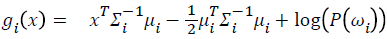→(13)

Naive Bayes classifier

The Naive Bayes classifier is a simple probabilistic classifier based on Bayes rule in Equation 14.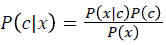→(14)→(15)

In the classifier, all the features are assumed as independent of each other within each class . Because of this, even if the features depend on each other, the classifier considers all the properties to independently affect the probability . One of the advantages of the Naïve Bayes classifier is that it only need mean and standard deviation of the variables in order to estimate the parameters for classification .→(16)→(17)

The decision rule for classification is choosing the more probable one according to the result of probability density function given below.→(18)

Support vector machines

Simple linear classifiers may be insufficient about classifying nonlinearly separable data or noisy data . Support vector machines can deal with nonlinear separable data by mapping the input data into a feature space of richer dimension and then makes classification as linear classification in that richer dimensional data. Linear, quadratic, polynomial, radial basis function type kernel functions are used for implementing this. One of the major works of the SVM is to find separating hyper planes which has the maximum margin to the support vectors. With the hyper plane that has maximum margin, the best separation boundary is found and most separation is made between the class members.

Neural networks

Neural networks are another classifier algorithm for classification works. Input patterns are mapped to outputs through successive layers as shown in Figure 3. Input layer has equal number of neurons to feature number and output layer has neurons to equal number of class. Also hidden layers can be added according to trial and error.

Outputs of the first hidden layer neurons are,

Outputs of the second hidden layer neurons are,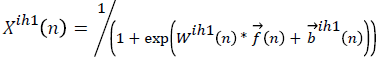→(19)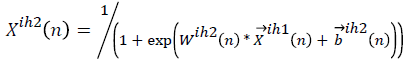→(20)

Outputs of the network are,→(21)

Here, Wih1 are weights of the input features to the first hidden layer and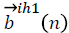are the biases of first hidden layer, Wih2 (n) are the weights from the first hidden layer to the second hidden layer,are the biases of the second hidden layer, Who (n) the weights from the second hidden layer to the output layer andare the biases of the output layer, values of the features, values of the outputs for the class index, and is training pattern index .

## Results

The system is tested by 8 persons. Firstly, the system is used by one person; the person trains the classifier with five different thumb motions, and tests how accurately the classifier classifies the movements. Then, the system is used interpersonally, one trains the classifier with five different thumb motions, and the others test how accurately the classifier classifies movements. The aim of interpersonal testing is to find the most robust or stable classifier algorithm. The performance results show the correct classification numbers of 100 trials. In addition, the computation time is evaluated for all classifiers, because this is a real-time application. Detailed evaluation is made for the classifiers because each classifier has various performance metrics means that Discriminant Analysis has various discriminant functions or Nearest Neighbor classifier has different distance rules and Support Vectors Machines have different Kernel Functions. All performance, standard deviation and computation time of the classifiers given in Table 1 for discriminant analysis, Table 2 for nearest neighbor, Table 3 for support vector machine, Table 4 for Naive Bayes and Table 5 for neural networks.

Ind Int Ind Int Ind Int Ind Int Ind Int
Perf. 92.75 56.571 75 33.714 91.625 59.571 87.625 45.714 73.5 33.857
STD 6.204 17.998 3.817 16.879 3.961 17.415 4.565 25.617 3.703 16.945
Comp. Time 0.0106 0.0066 0.0011 0.0014 0.0025

Table 1: Results of discriminant analysis.

KNN Rule/Distance Euclidean City block Cosine Correlation
Ind Int Ind Int Ind Int Ind Int
Performance Nearest 82.625 73.142 82.625 66.285 81.750 59.285 81.875 59.571
STD 2.973 4.525 3.020 9.304 3.693 8.518 3.522 8.182
Comp. Time 0.0097 0.0081 0.0050 0.0047
Performance Random 82.375 70.142 82.250 67.142 80.625 59.285 81.250 59.428
STD 4.068 6.618 3.195 7.883 3.292 8.769 3.453 8.791
Comp. Time 0.0083 0.0091 0.0040 0.0043
Performance Consensus 42.625 28.428 43.125 27.714 39.625 22.000 39.500 22.000
STD 6.045 9.015 6.1281 9.268 12.949 10.739 12.501 10.535
Comp. Time 0.0086 0.0087 0.0047 0.0047

Table 2: Results of k-nearest neighbor.

Ind Int Ind Int Ind Int Ind Int
Performance 78.375 40.000 77.000 39.428 66.25 34.714 29.375 20.000
STD 6.696 13.868 4.407 12.081 5.175 9.638 3.814 0.000
Comp. Time 0.9737 1.4295 1.1649 0.2832

Table 3: Results of support vector machine.

Naive Bayes Gaussian Kernel
Ind Int Ind Int
Performance 76.250 44.857 87.000 44.857
STD 9.837 24.044 5.631 24.155
Comp. Time 0.0075 0.1044

Table 4: Results of Naive Bayes.

NN Feed forward back propagation network, no hidden layer, transfer functions are “tansig” for input and output layer. Training function is “trainlm”
Ind Int
Performance 74 63.142
STD 10.823 13.056
Comp. Time 0.0159

Table 5: Results of neural networks.

Figure 4 is a box-whisker plot of the performances of the classifiers. This figure is a good summary of the results of each classifier. On each box the central mark is median, edges of the box are the 1st and 3rd quartiles and the whiskers extend to the minimum and maximum data points. The same classifiers colored with the same color, "Ind" represents the individual, meaning train and test belong to the same person, "Int" represents the interpersonal, meaning train and test belong to different people. The performance of the classifier algorithms is highly related to the properties of the data to be classified. The specific relationship between data and the appropriate classifier is still undefined. Therefore, there is no assumption about any classification problem and the best classifier. The trial and error technique is still used to determine the best classifier for a given classification problem. In this study, we used the trial and error technique to determine the best classifier to classify joystick control movements.

Figure 4: Compare of the most successful classification algorithms both individual and interpersonal test.

According to results, LDA is the best classifier for individually usage and KNN is the best classifier for interpersonally usage as seen in Figure 4 and exact values are in Table 1 for LDA and Table 2 for KNN. These results prove that joystick movements can be linearly separated during personal use. We make this assumption because the decision boundary of the LDA is linear and the highest classification accuracy for personal use belongs to the LDA. The average classification success of LDA is 92.75%. There are also cases where the classification success is 100% when looking at the top of whisker plot of LDA_Ind. Classifiers with nonlinear decision boundaries such as QDA and quadratic core SVM (SVM_Q) has a smaller accuracy than linear models such as LDA or SVM. Here, the imitation ability of a person plays a vital role in the name of performance, so the movement of the thumb must be similar to or the same as that performed while training the classifier. For this reason, the performance of the system is experientially increasing; Beginners often give worse results than experienced people who have used the system over and over because beginners cannot fully imitate the training movements during the test. This situation is affecting the standard deviation.

On the other hand, the results show that KNN is the best classifier algorithm for interpersonal use and has an average performance of 73.142%. The performance of the LDA is unstable and worse when compared to the KNN in interpersonal use. This proves that our separating boundary is changed from linear to non-linear. The main reason for this change is that electrode placement is not exactly the same between users and the EMG signal level is different for each user. This makes the decision boundary highly non-linear. We can expect good results from nonlinear classifiers such as QDA or SVM_Q, but they do not give good results. The reason is that these classifier algorithms cannot model the highly nonlinear decision boundary very well. Because these classifiers can overcome moderate nonlinear states. KNN performs better than other classifiers; Because KNN is a nonparametric classifier, no assumption is made about the shape of the decision boundary in KNN. In addition, other nonlinear classifiers accept a global decision boundary, but the decision boundaries of the KNN are local for each sample. No calculations are made when new data is added to the old data in KNN, all calculations are done during the test. Because of this speciality KNN can outperform other techniques when the decision boundary is highly non-linear. Another advantage of KNN is that it is robust against deviation compared to others in both individual and interpersonal use, as shown in Figure 4.

When the classifier computation time is compared, both KNN and LDA are the fastest algorithms compared to the others. Computation time is important because this system is a real time system.

## Conclusion

A user interface is designed for classification of the joystick gesture of thumb. The interface was used by 8 volunteer participants and the findings obtained by performing 64 experiments in total were explained in the results section. As a conclusion LDA is the best performance classifier for thumb finger gesture classification but KNN is the most robust classifier. KNN and LDA may be combined for future studies. The system can be regarded as a reliable wearable joystick since the average performance is over 92%. When looking at the upper extreme of the whisker of the LDA_Ind classifier in Figure 4, it is possible to achieve a 100% success rate even though the average performance of the system is 92%. It is always up to the user to get this 100% success rate. It is known that EMG signals are caused by contraction of muscles. For this reason, muscle contraction of the thumb must be close to each other or must be the same during training and testing. For same contractions, finger should be forced in same quantities in training and testing. As the user practices, system performance improves. The EMG device we use is singlechannel and the performance will be over 92% with the use of multi-channel EMG device.

## Acknowledgement

This work is supported by the Bozok University’s 2015FBE/ T166 coded BAP project.